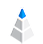# The Mystery of Circle Visualization

Data visualization is a very important and useful tool during the daily decision-making processes. Why? Because the human brain can process graphs and charts much more easily than spreadsheets or databases. Therefore visual presentations could be very informative if we use them in the right way. There are many of reccommendations about the right use of several visualizations, but these opinions sometimes conflict with each other.

In this post, we will try to find the answer bubble charts. A bubble chart is a modified version of a simple scatter plot with which you can visualize your numerical data along two angles. In the case of bubble charts, we replace the data points with circles or bubbles of a given size which, means an additional dimension. Therefore, you can use a third measurement that could give you deeper insights into your data.

If someone asks you to choose a circle that is twice as big a previous circle, which selection method would you use? Would you choose the circle with double the area or the one with double radius? How do people identify the size of a circle? Check out our test, and you will get the answers to these questions.

## The Experiment

In this section, we will show you how we tested our users in this research study.

In the picture below, you can see three circles with their appropriate properties. Circle 1 is the reference circle, Circle 2 circle has twice the area of the first, and Circle 3 has twice the radius of the first.In the first phase of the research study, we had to develop an environment in which to conduct them. The technical background is irrelevant, so we will not bore you with it.

During the development we had the following expectations:

• The reference circle has to be on the left side.
• The reference circle should not be too small, the human eye should be able to see it easily.
• On the right side, we will show ten circles with randomly generated properties (position, size, fill). The biggest circle’s radius cannot have more than three times the radius of the reference circle.

The second phase was the test phase. The users picked the circle that they thought was the correct answer to the question. In the picture below, you will see the test environment from which we gathered our data.Users picked 100 circles during the test period. The results are shown in the scatter plot below.Two gray reference lines show the following:

• The radius is twice as big (y=2x)
• The area is twice as big (y=√2x)

The following comparison illustrates the differences between the two most different data sets. (Click to zoom)The charts clearly show that the method of choosing can be completely different for each user.

For example, User 4 usually chose the circles that had an area that was twice the size of the reference circles. However, User 2 usually chose the circles that a radius that was twice the size of the reference circles (and thus, an area that was at least four times bigger).

## Conclusion

If we want to visualize a quantitative dataset via circles, we have to indicate the method of the drawing to the user. It is important to communicate this information because people think differently some think about the area while others think about radius. The area-based approach to a circle visualization is a bit more comfortable because it results in spacious and more attractive visualization.

Several things may influence the thinking of people in this situation, such as visual effects (distance from the reference circle), logic skills, fields of interest, and technical skills.

Connect your data and start the exploration